# Difference between revisions of "Prefix sum array and difference array"

Given an array of numbers, we can construct a new array by replacing each element by the difference between itself and the previous element, except for the first element, which we simply ignore. This is called the difference array, because it contains the first differences of the original array. For example, the difference array of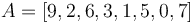$A = [9, 2, 6, 3, 1, 5, 0, 7]$ is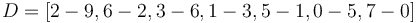$D = [2-9, 6-2, 3-6, 1-3, 5-1, 0-5, 7-0]$, or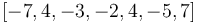$[-7, 4, -3, -2, 4, -5, 7]$. Evidently, the difference array can be computed in linear time from the original array.

We can run this process in reverse: that is, given a difference array and the initial element of the original array, we can reconstruct the original array. To do this, we simply scan from left to right, and accumulate as we go along, giving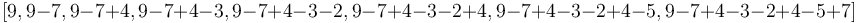$[9, 9-7, 9-7+4, 9-7+4-3, 9-7+4-3-2, 9-7+4-3-2+4, 9-7+4-3-2+4-5, 9-7+4-3-2+4-5+7]$. The reader should perform this calculation and confirm that this is indeed equal to$A = [9, 2, 6, 3, 1, 5, 0, 7]$. This reverse process can also be done in linear time, as each element in$A$ is the sum of the corresponding element in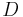$D$ and the previous element in$A$. We say that$A$ is a prefix sum array of$D$. However, it is not the only prefix sum array of$D$, because knowing$D$ alone does not give us enough information to reconstruct$A$; we also needed to know the initial element of$A$. If we assumed that this were -3, for example, instead of 9, we would obtain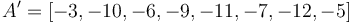$A' = [-3, -10, -6, -9, -11, -7, -12, -5]$.$D$ is the difference array of both$A$ and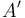$A'$. Both$A$ and$A'$ are prefix sum arrays of$D$.

## Analogy with calculus

These two processes—computing the difference array, and computing a prefix sum array—are the discrete equivalents of differentiation and integration in calculus, which operate on continuous domains:

• Differentiation and integration are reverses. Computing the difference array and computing a prefix sum array are reverses.
• A function can only have one derivative, but an infinite number of antiderivatives. An array has only one difference array, but an infinite number of prefix sum arrays.
• However, if we know the value of a function over an interval of the real line and we are given some real number, we can find one unique antiderivative of this function which attains this real value at the left end of the interval. Likewise, if we are given an array, and we are told the initial element of one of its prefix sum arrays, we can reconstruct the entire prefix sum array.
• When we take the difference array, we shorten the array by one element, since we destroy information about what the initial element was. On the other hand, when we take a prefix sum array, we lengthen the array by one element, since we introduce information about what the initial element is to be. Likewise, when we take the derivative of a function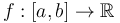$f:[a,b]\to\mathbb{R}$ on a closed interval, we obtain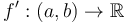$f':(a,b)\to\mathbb{R}$ (open interval), and we destroy the information of what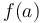$f(a)$ was; conversely, if we are given$f':(a,b)\to\mathbb{R}$ (open interval), and the value of$f(a)$ is also told to us, we obtain its antiderivative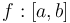$f:[a,b]$ (closed interval).
• All possible prefix sum arrays differ only by an offset from each other, in the sense that one of them can be obtained by adding a constant to each entry of the other. For example, the two prefix sum arrays shown for$A$ above differ by 12 at all positions. Likewise, all possible antiderivatives differ from each other only by a constant (the difference between their constants of integration).

Because of these similarities, we will speak simply of differentiating and integrating arrays. An array can be differentiated multiple times, but eventually it will shrink to length 0. An array can be integrated any number of times. Differentiating$k$ times and integrating$k$ times are still reverse processes.

## Use of prefix sum array

The Fundamental Theorem of Calculus also has an analogue, which is why the prefix sum array is so useful. If we assume both the original array and its prefix sum array are zero-indexed (or both one-indexed), for example,$A = [9, 2, 6, 3, 1, 5, 0, 7]$ where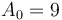$A_0 = 9$ and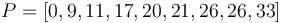$P = [0, 9, 11, 17, 20, 21, 26, 26, 33]$ (a possible prefix sum array of$A$, where we have arbitrarily chosen 0 as the initial element), where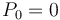$P_0 = 0$, then we can add up any range of elements in$A$ by taking the difference of two elements in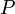$P$. In particular,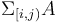$\Sigma_{[i,j)}A$, the notation we will use for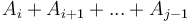$A_i + A_{i+1} + ... + A_{j-1}$, is equal to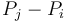$P_j - P_i$. (Note the use of the half-open interval.) For example,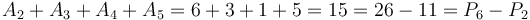$A_2 + A_3 + A_4 + A_5 = 6 + 3 + 1 + 5 = 15 = 26 - 11 = P_6 - P_2$. This property holds no matter what we choose as the initial element of$P$, because it will always cancel out of the calculation, just as we can use any antiderivative of a function to compute an integral (which is like summing a function across an entire interval) by taking the difference between the antiderivative's values at the endpoints, because the constant of integration will always cancel out. This makes the prefix sum array a useful item to precompute; once we have computed it, which only takes linear time, we can sum any range of the original array in constant time, simply by taking the difference of two values in the prefix sum array.

### Example: Partial Sums (SPOJ)

Computing the prefix sum array is rarely the most difficult part of a problem. Instead, the prefix sum array is kept on hand because the algorithm to solve the problem makes frequent reference to range sums.

For example, consider the problem Partial Sums. Here we are given an array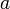$a$ of up to 105 integers, a modulus$P$ and a remainder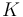$K$. The problem asks us to determine the minimal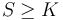$S \geq K$ such that there exists a nonempty slice of$a$ whose sum is congruent to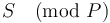$S\pmod{P}$.

To solve this, we will first transform array$a$ into its prefix sum array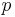$p$. Then, the problem reduces to finding the minimal$S \geq K$ such that there exist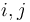$i,j$ with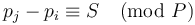$p_j - p_i \equiv S\pmod{P}$, since the difference between a pair of elements of$p$ is the sum of a range in$a$. (We do not allow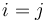$i = j$ because then the corresponding range from$a$ would be empty.)

This can be solved by iterating through the prefix sum array from left to right, trying out every possible value of$j$. We assume that all values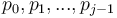$p_0, p_1, ..., p_{j-1}$ have already been added to an ordered dynamic set. If we intend to find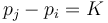$p_j - p_i = K$, then we must have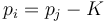$p_i = p_j - K$. If we intend to find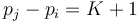$p_j - p_i = K+1$, then we must have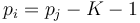$p_i = p_j - K - 1$; and so on up to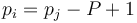$p_i = p_j - P + 1$; so we want to identify the first element in the sequence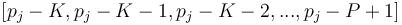$[p_j-K, p_j-K-1, p_j-K-2, ..., p_j-P+1]$ which is congruent to something already in the set. (We can do this using set operations; the details are left as an exercise to the reader.) Doing so will allow us to compute the optimal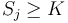$S_j \geq K$; the smallest modular range sum at least$K$ that ends at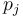$p_j$ (i.e., ends at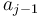$a_{j-1}$). After we've done this, we add$p_j$ to our set, and move on to the next iteration. Finally,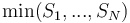$\min(S_1, ..., S_N)$ is the answer. Overall time is$O(N \log N)$.